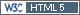Hi Angus,

» We have completed a dose proportionality study using the lowest strength to the highest strength of a modified release formulation of a class I drug. The approach used was the usual cross-over design with 20 subjects (AB, BA) and dose normalized the PK parameters Cmax and AUC0-T prior to bioequivalence. No problem we are BE comfortably within the limits (0.8-1.25).

Testing only two levels for dose proportionality is somewhat unconventional.

» Another worker has used the power model with the same data set for dose proportionality […]

Does it look like this (high dose = 8× low dose)?» The point estimates are much the same as my approach, […]

Numbers? AUC only – Cmax is of limited value in DP.

» […] but he quotes 98% CI.

Smells of Bonferroni’s adjustment for four simultaneous (and independent) tests in order to control the familywise error rate: 100(1–2α/4) = 97.5% CI and FWER 1–(1–α)4 = 0.0491.
In the power model we have only two parameters (the coefficient α and the exponent β: E[D] = α·D β (independent from the number of dose levels tested) and we are interested only in β. I don’t see why a multiplicity-adjustment was done.

» His CI values are also within the lmits.

How did he get a CI of β? With two dose levels we have zero degrees of freedom for a model with two para­meters.

Before we can discuss variances, we need more information.

Cheers,
Helmut SchützThe quality of responses received is directly proportional to the quality of the question asked. ☼
Science QuotesIng. Helmut Schütz